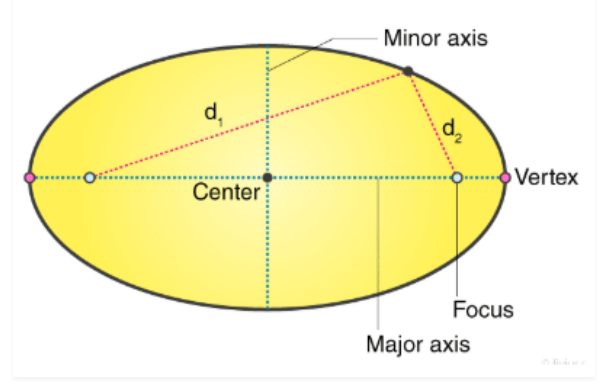# What does ellipse mean?

An ellipse is the locus of all those points in a plane such that the sum of their distances from two fixed points in the plane, is constant. The fixed points are known as the foci which are surrounded by the curve.

• The ellipse is one of the conic sections, that is produced, when a plane cuts the cone at an angle with the base. If the cone is intersected by the plane, parallel to the base, then it forms a circle.
• In geometry, an ellipse is a two-dimensional shape, that is defined along its axes. An ellipse is formed when a cone is intersected by a plane at an angle with respect to its base.
• It has two focal points. The sum of the two distances to the focal point, for all the points in curve, is always constant.
• A circle is also an ellipse, where the foci are at the same point, which is the center of the circle.

## Properties of ellipse

• Ellipse has two focal points, also called foci.
• The fixed distance is called a directrix.
• The eccentricity of ellipse lies between 0 to 1. 0≤e<1
• The total sum of each distance from the locus of an ellipse to the two focal points is constant
• Ellipse has one major axis and one minor axis and a center.(1)(0)